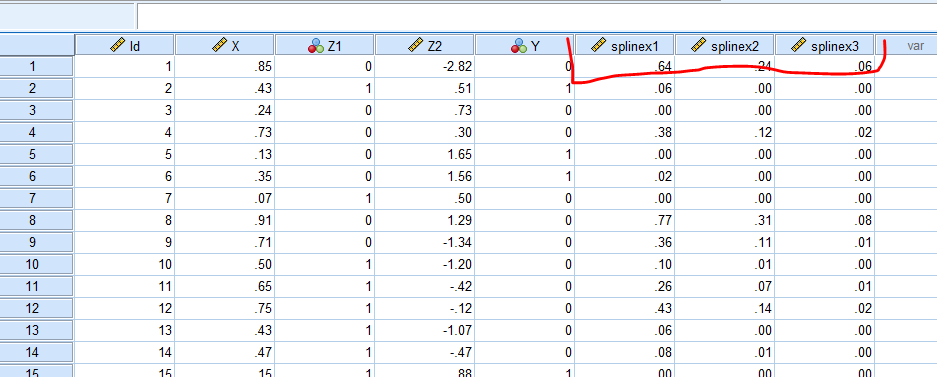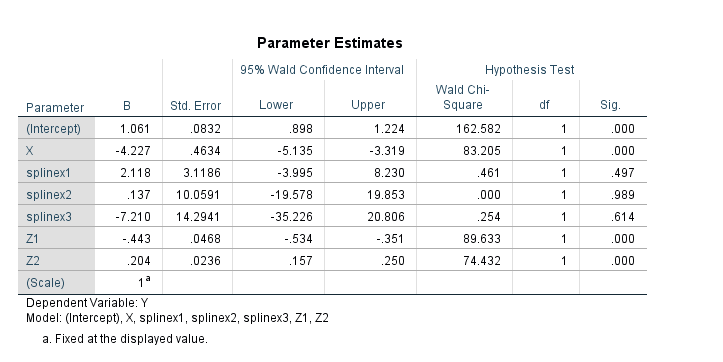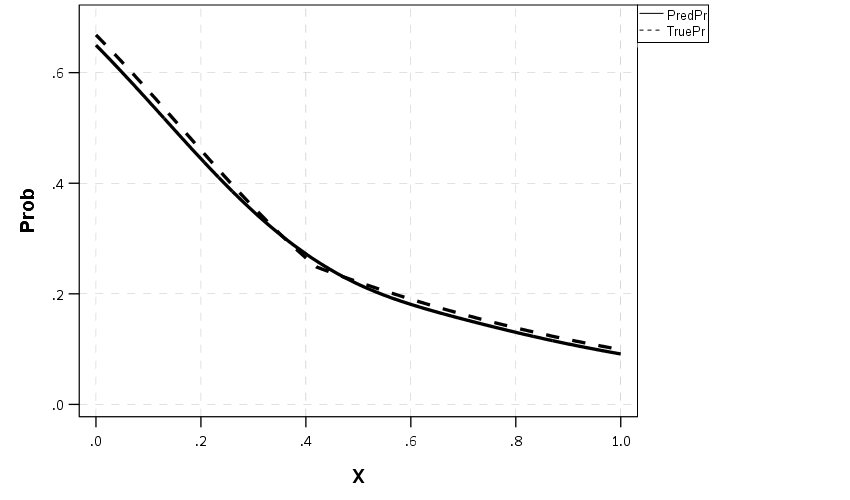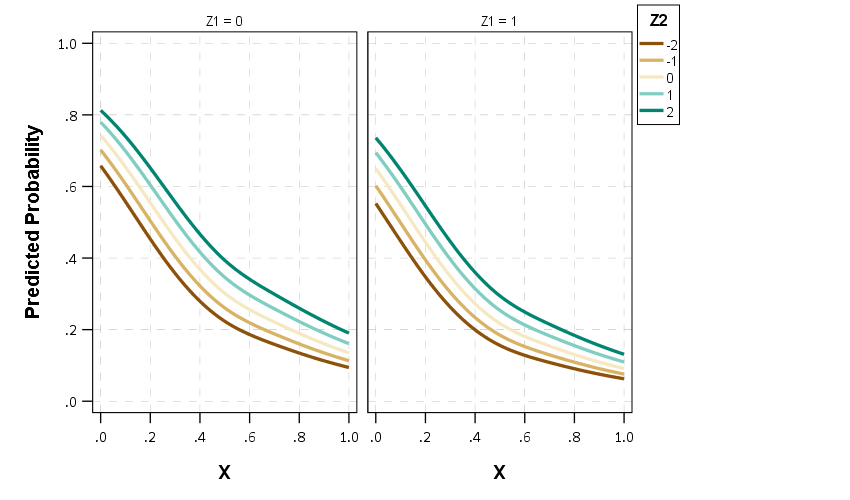# Graphing Spline Predictions in SPSS

I might have around 10 blog posts about using splines in regression models – and you are about to get another. Instead of modeling non-linear effects via polynomial terms (e.g. including x^2, x^3 in a model, etc.), splines are a much better default procedure IMO. For a more detailed mathy exposition on splines and a walkthrough of the functions, see my class notes.

So I had a few questions about applying splines in generalized linear models and including control variables in my prior post (on a macro to estimate the spline terms). These include can you use them in different types of generalized linear models (yes), can you include other covariates into the model (yes). For either of those cases, interpreting the splines are more difficult though. I am going to show an example here of how to do that.

Additionally I have had some recent critiques of my paper on CCTV decay effects. One is that the locations of the knots we chose in that paper is arbitrary. So while that is true, one of the reasons I really like splines is that they are pretty robust – you can mis-specify the knot locations, and if you have enough of them they will tend to fit quite a few non-linear functions. (Also a note on posting pre-prints, despite being rejected twice and under review for around 1.5 years, it has over 2k downloads and a handful of citations. The preprint has more downloads than my typical published papers do.)

So here I am going to illustrate these points using some simulated data according to a particular logistic regression equation. So I know the true effect, and will show how mis-located spline knots still recovers the true effect quite closely. This example is in SPSS, and uses my macro on estimating spline basis.

## Generating Simulated Data

So first in SPSS, I define the location where I am going to save my files. Then I import my Spline macro.

``````* Example of splines for generalized linear models
* and multiple variables.

DATASET CLOSE ALL.
OUTPUT CLOSE ALL.

* Spline Macro.
FILE HANDLE macroLoc /name = "C:\Users\andre\OneDrive\Desktop\Spline_SPSS_Example".
INSERT FILE = "macroLoc\MACRO_RCS.sps".``````

Second, I create a set of synthetic data, in which I have a linear changepoint effect at x = 0.42. Then I generate observations according to a particular logistic regression model, with not only the non-linear X effects, but also two covariates Z1 (a binary variable) and Z2 (a continuous variable).

``````*****************************************************.
* Synthetic data.
SET SEED = 10.
INPUT PROGRAM.
LOOP Id = 1 to 10000.
END CASE.
END LOOP.
END file.
END INPUT PROGRAM.
DATASET NAME Sim.

COMPUTE X = RV.UNIFORM(0,1).
COMPUTE #Change = 0.42.
DO IF X <= #Change.
COMPUTE XDif = 0.
ELSE.
COMPUTE XDif = X - #Change.
END IF.
COMPUTE Z1 = RV.BERNOULLI(0.5).
COMPUTE Z2 = RV.NORMAL(0,1).

DEFINE !INVLOGIT (!POSITIONAL  !ENCLOSE("(",")") )
1/(1 + EXP(-!1))
!ENDDEFINE.

*This is a linear changepoint at 0.42, other variables are additive.
COMPUTE ylogit = 1.1 + -4.3*x + 2.4*xdif + -0.4*Z1 + 0.2*Z2.
COMPUTE yprob = !INVLOGIT(ylogit).
COMPUTE Y = RV.BERNOULLI(yprob).
*These are variables you won't have in practice.
ADD FILES FILE =* /DROP ylogit yprob XDif.
FORMATS Id (F9.0) Y Z1 (F1.0) X Z2 (F3.2).
EXECUTE.
*****************************************************.``````

## Creating Spline Basis and Estimating a Model

Now like I said, the correct knot location is at `x = 0.42`. Here I generate a set of regular knots over the x input (which varies from 0 to 1), at not the exact true value for the knot.

``!rcs x = X loc = [0.1 0.3 0.5 0.7 0.9].``

Now if you look at your dataset, there are 3 new `splinex?` variables. (For restricted cubic splines, you get `# of knots - 2` new variables, so with 5 knots you get 3 new variables here.)We are then going to use those new variables in a logistic regression model. We are also going to save our model results to an xml file. This allows us to use that model to score a different dataset for predictions.

``````GENLIN Y (REFERENCE=0) WITH X splinex1 splinex2 splinex3 Z1 Z2
/MODEL X splinex1 splinex2 splinex3 Z1 Z2
/OUTFILE MODEL='macroLoc\LogitModel.xml'. ``````

And if we look at the coefficients, you will see that the coefficients look offhand very close to the true coefficients, minus splinex2 and splinex3. But we will show in a second that those effects should be of no real concern.## Generating New Data and Plotting Predictions

So you should do this in general with generalized linear models and/or non-linear effects, but to interpret spline effects you can’t really look at the coefficients and know what those mean. You need to make plots to understand what the non-linear effect looks like.

So here in SPSS, I create a new dataset, that has a set of regularly sampled locations along X, and then set the covariates `Z1=1` and `Z2=0`. These set values you may choose to be at some average, such as mean, median, or mode depending on the type of covariate. So here since Z1 can only take on values of 0 and 1, it probably doesn’t make sense to choose 0.5 as the set value. Then I recreate my spline basis functions using the exact sample macro call I did earlier.

``````INPUT PROGRAM.
LOOP #xloc = 0 TO 300.
COMPUTE X = #xloc/300.
END CASE.
END LOOP.
END FILE.
END INPUT PROGRAM.
DATASET NAME Fixed.
COMPUTE Z1 = 1.
COMPUTE Z2 = 0.
EXECUTE.
DATASET ACTIVATE Fixed.

*Redoing spline variables.
!rcs x = X loc = [0.1 0.3 0.5 0.7 0.9].``````

Now in SPSS, we score this dataset using our prior model xml file we saved. Here this generates the predicted probability from our logistic model.

``````MODEL HANDLE NAME=LogitModel FILE='macroLoc\LogitModel.xml'.
COMPUTE PredPr = APPLYMODEL(LogitModel, 'PROBABILITY', 1).
EXECUTE.
MODEL CLOSE NAME=LogitModel.``````

And to illustrate how close our model is, I generate what the true predicted probability should be based on our simulated data.

``````*Lets also do a line for the true effect to show how well it fits.
COMPUTE #change = 0.42.
DO IF X <= #change.
COMPUTE xdif = 0.
ELSE.
COMPUTE xdif = (X - #change).
END IF.
EXECUTE.
COMPUTE ylogit = 1.1 + -4.3*x + 2.4*xdif + -0.4*Z1 + 0.2*Z2.
COMPUTE TruePr = !INVLOGIT(ylogit).
FORMATS TruePr PredPr X (F2.1).
EXECUTE.``````

And now we can put these all into one graph.

``````DATASET ACTIVATE Fixed.
GGRAPH
/GRAPHDATASET NAME="graphdataset" VARIABLES=X PredPr TruePr
/FRAME INNER=YES
/GRAPHSPEC SOURCE=INLINE.
BEGIN GPL
SOURCE: s=userSource(id("graphdataset"))
DATA: X=col(source(s), name("X"))
DATA: PredPr=col(source(s), name("PredPr"))
DATA: TruePr=col(source(s), name("TruePr"))
GUIDE: axis(dim(1), label("X"))
GUIDE: axis(dim(2), label("Prob"))
SCALE: cat(aesthetic(aesthetic.shape), map(("PredPr",shape.solid),("TruePr",shape.dash)))
ELEMENT: line(position(X*PredPr), shape("PredPr"))
ELEMENT: line(position(X*TruePr), shape("TruePr"))
END GPL.``````So you can see that even though I did not choose the correct knot location, my predictions are nearly spot on with what the true probability should be.

## Generating Predictions Over Varying Inputs

So in practice you can do more complicated models with these splines, such as allowing them to vary over different categories (e.g. interactions with other covariates). Or you may simply want to generate predicted plots such as above, but have a varying set of inputs. Here is an example of doing that; for Z1 we only have two options, but for Z2, since it is a continuous covariate we sample it at values of -2, -1, 0, 1, 2, and generate lines for each of those predictions.

``````*****************************************************.
* Can do the same thing, but vary Z1/Z2.

DATASET ACTIVATE Sim.
DATASET CLOSE Fixed.

INPUT PROGRAM.
LOOP #xloc = 0 TO 300.
LOOP #z1 = 0 TO 1.
LOOP #z2 = -2 TO 2.
COMPUTE X = #xloc/300.
COMPUTE Z1 = #z1.
COMPUTE Z2 = #z2.
END CASE.
END LOOP.
END LOOP.
END LOOP.
END FILE.
END INPUT PROGRAM.
DATASET NAME Fixed.
EXECUTE.
DATASET ACTIVATE Fixed.

*Redoing spline variables.
!rcs x = X loc = [0.1 0.3 0.5 0.7 0.9].

MODEL HANDLE NAME=LogitModel FILE='macroLoc\LogitModel.xml'.
COMPUTE PredPr = APPLYMODEL(LogitModel, 'PROBABILITY', 1).
EXECUTE.
MODEL CLOSE NAME=LogitModel.

FORMATS Z1 Z2 (F2.0) PredPr X (F2.1).
VALUE LABELS Z1
0 'Z1 = 0'
1 'Z1 = 1'.
EXECUTE.

*Now creating a graph of the predicted probabilities over various combos.
*Of input variables.
DATASET ACTIVATE Fixed.
GGRAPH
/GRAPHDATASET NAME="graphdataset" VARIABLES=X PredPr Z1 Z2
/FRAME INNER=YES
/GRAPHSPEC SOURCE=INLINE.
BEGIN GPL
SOURCE: s=userSource(id("graphdataset"))
DATA: X=col(source(s), name("X"))
DATA: PredPr=col(source(s), name("PredPr"))
DATA: TruePr=col(source(s), name("TruePr"))
DATA: Z1=col(source(s), name("Z1"), unit.category())
DATA: Z2=col(source(s), name("Z2"), unit.category())
COORD: rect(dim(1,2), wrap())
GUIDE: axis(dim(1), label("X"))
GUIDE: axis(dim(2), label("Predicted Probability"))
GUIDE: axis(dim(3), opposite())
GUIDE: legend(aesthetic(aesthetic.color), label("Z2"))
SCALE: cat(aesthetic(aesthetic.color), map(("-2",color."8c510a"),("-1",color."d8b365"),
("0",color."f6e8c3"), ("1",color."80cdc1"), ("2",color."018571")))
ELEMENT: line(position(X*PredPr*Z1), color(Z2))
END GPL.
*****************************************************.``````So between all of these covariates, the form of the line does not change much (as intended, I simulated the data according to an additive model).

If you are interested in drawing more lines for Z2, you may want to use a continuous color scale instead of a categorical one (see here for a similar example).